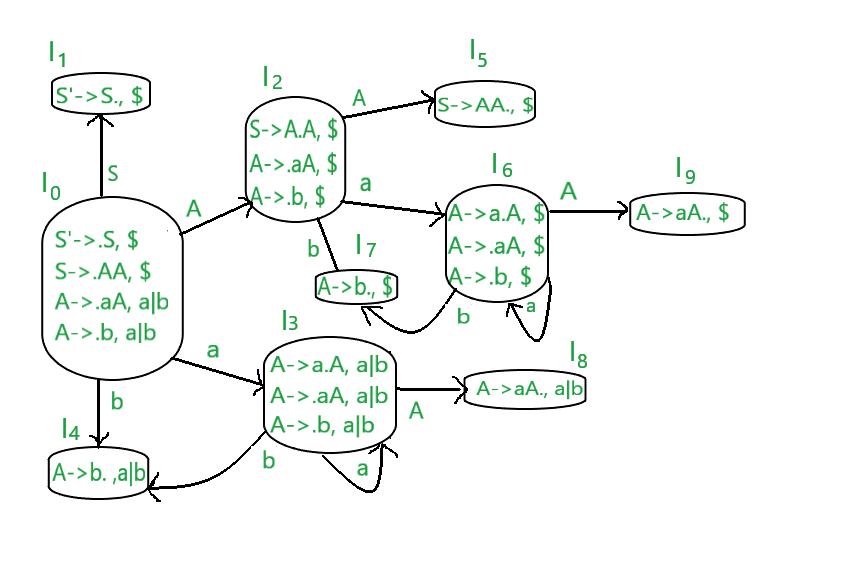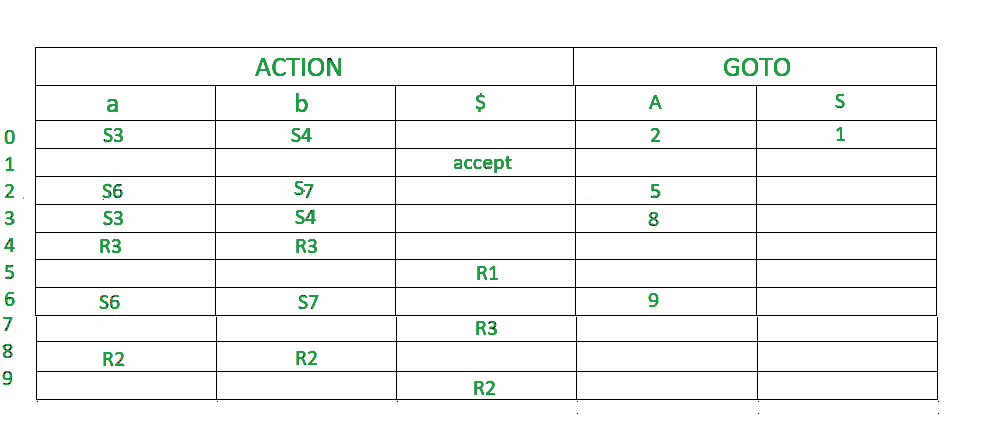Open in App
Not now

# CLR Parser (with Examples)

• Difficulty Level : Basic
• Last Updated : 29 Oct, 2022

LR parsers :
It is an efficient bottom-up syntax analysis technique that can be used to parse large classes of context-free grammar is called LR(k) parsing.
L stands for the left to right scanning
R stands for rightmost  derivation in reverse
k stands for no. of input symbols of lookahead

• It recognizes virtually  all programming language constructs for which CFG can be written
• It is able to detect syntactic errors
• It is an efficient non-backtracking shift reducing parsing method.

Types of LR parsing methods :

1. SLR
2. CLR
3. LALR

CLR Parser :
The CLR parser stands for canonical LR parser.It is a more powerful LR parser.It makes use of lookahead symbols. This method uses a large set of items called LR(1) items.The main difference between LR(0)  and LR(1) items is that, in LR(1) items, it is possible to carry more information in a state, which will rule out useless reduction states.This extra information is incorporated into the state by the lookahead symbol. The general syntax becomes  [A->∝.B, a ]
where A->∝.B is the production and a is a terminal or right end marker \$
LR(1) items=LR(0) items + look ahead

CASE 1 –

`A->∝.BC, a `

Suppose this is the 0th production.Now, since ‘ . ‘ precedes B,so we have to write B’s productions as well.

`B->.D [1st production]`

Suppose this is B’s production. The look ahead of this production is given as we look at previous productions ie 0th production. Whatever is after B, we find FIRST(of that value) , that is the lookahead of 1st production.So,here in 0th production, after B, C is there. assume FIRST(C)=d, then 1st production become

`B->.D, d`

CASE 2 –
Now if the 0th production was like this,

`A->∝.B, a `

Here, we can see there’s nothing after B. So the lookahead of 0th production will be the lookahead of 1st production. ie-

`B->.D, a`

CASE 3 –
Assume a production A->a|b

```A->a,\$ [0th production]
A->b,\$ [1st production]```

Here, the 1st production is a part of the previous production, so the lookahead will be the same as that of its previous production.
These are the 2 rules of look ahead.

Steps for constructing CLR parsing table :

1. Writing augmented grammar
2. LR(1) collection of items to be found
3. Defining 2 functions: goto[list of terminals] and action[list of non-terminals] in the CLR parsing table

EXAMPLE
Construct a CLR parsing table for the given context-free grammar

```S-->AA
A-->aA|b```

Solution :
STEP 1 – Find augmented grammar

The augmented grammar of the given grammar is:-

```S'-->.S ,\$   [0th production]
S-->.AA ,\$ [1st production]
A-->.aA ,a|b [2nd production]
A-->.b ,a|b [3rd production]```

Let’s apply the rule of lookahead to the above productions

• The initial look ahead is always \$
• Now, the 1st production came into existence because of ‘ . ‘ Before ‘S’ in 0th production.There is nothing after ‘S’, so the lookahead of 0th production will be the lookahead of 1st production. ie:  S–>.AA ,\$
• Now, the 2nd production came into existence because of ‘ . ‘ Before ‘A’ in the 1st production.After ‘A’, there’s  ‘A’. So, FIRST(A) is a,b
Therefore,the look ahead for the 2nd production becomes a|b.
• Now, the 3rd production is a part of the 2nd production.So, the look ahead will be the same.

STEP 2 – Find LR(1) collection of items
Below is the figure showing the LR(1) collection of items. We will understand everything one by one.The terminals of this grammar are {a,b}
The non-terminals of this grammar are {S,A}

RULE-

1. If any non-terminal has ‘ . ‘ preceding it, we have to write all its production and add ‘ . ‘ preceding each of its production.
2. from each state to the next state, the ‘ . ‘ shifts to one place to the right.
3. All the rules of lookahead apply here.
• In the figure, I0 consists of augmented grammar.
• Io goes to I1 when  ‘ . ‘ of 0th production is shifted towards the right of S(S’->S.). This state is the accept state . S is seen by the compiler. Since I1 is a part of the 0th production, the lookahead is the same ie \$
• Io goes to I2 when  ‘ . ‘ of 1st production is shifted towards right (S->A.A) . A is seen by the compiler. Since I2 is a part of the 1st production, the lookahead is the same i.e. \$.
• I0 goes to I3 when  ‘ . ‘ of the 2nd production is shifted towards right (A->a.A) . a is seen by the compiler. Since I3 is a part of the 2nd production, the lookahead is the same ie a|b.
• I0 goes to I4 when  ‘ . ‘ of the 3rd production is shifted towards right (A->b.) . b is seen by the compiler. Since I4 is a part of the 3rd production, the lookahead is the same i.e. a | b.
• I2 goes to I5 when  ‘ . ‘ of 1st production is shifted towards right (S->AA.) . A is seen by the compiler. Since I5 is a part of the 1st production, the lookahead is the same i.e. \$.
• I2 goes to I6 when  ‘ . ‘ of 2nd production is shifted towards the right (A->a.A) . A is seen by the compiler. Since I6 is a part of the 2nd production, the lookahead is the same i.e. \$.
• I2 goes to I7 when  ‘ . ‘ of 3rd production is shifted towards right (A->b.) . A is seen by the compiler. Since I6 is a part of the 3rd production, the lookahead is the same i.e. \$.
• I3 goes to I3 when  ‘ . ‘ of the 2nd production is shifted towards right (A->a.A) . a is seen by the compiler. Since I3 is a part of the 2nd production, the lookahead is the same i.e. a|b.
• I3 goes to I8 when  ‘ . ‘ of 2nd production is shifted towards the right (A->aA.) . A is seen by the compiler. Since I8 is a part of the 2nd production, the lookahead is the same i.e. a|b.
• I6 goes to I9 when  ‘ . ‘ of 2nd production is shifted towards the right (A->aA.) . A is seen by the compiler. Since I9 is a part of the 2nd production, the lookahead is the same i.e. \$.
• I6 goes to I6 when  ‘ . ‘ of the 2nd production is shifted towards right (A->a.A) . a is seen by the compiler. Since I6 is a part of the 2nd production, the lookahead is the same i.e. \$.
• I6 goes to I7 when  ‘ . ‘ of the 3rd production is shifted towards right (A->b.) . b is seen by the compiler. Since I6 is a part of the 3rd production, the lookahead is the same ie \$.

STEP 3-  defining 2 functions:goto[list of terminals] and action[list of non-terminals] in the parsing table.Below is the CLR parsing table• \$ is by default a non terminal which takes accepting state.
• 0,1,2,3,4,5,6,7,8,9 denotes I0,I1,I2,I3,I4,I5,I6,I7,I8,I9
• I0 gives A in I2, so 2 is added to the A column and 0 row.
• I0 gives S in I1,so 1 is added to the S column and 1st row.
• similarly  5 is written in  A column and 2nd  row, 8 is written in A column and 3rd row, 9 is written in A column and 6th row.
• I0 gives a in I3, so S3(shift 3) is added to a column and 0 row.
• I0 gives b in I4, so S4(shift 4) is added to the b column and 0 row.
• Similarly, S6(shift 6) is added on ‘a’ column and 2,6 row ,S7(shift 7) is added on b column and 2,6 row,S3(shift 3) is added on ‘a’ column and 3 row ,S4(shift 4) is added on b column and 3 row.
• I4 is reduced as ‘ . ‘ is at the end. I4 is the 3rd production of grammar. So write r3(reduce 3) in lookahead columns. The lookahead of I4 are a and b, so write R3 in a and b column.
• I5 is reduced as ‘ . ‘ is at the end. I5 is the 1st production of grammar. So write r1(reduce 1) in lookahead columns. The lookahead of I5 is \$ so write R1 in \$ column.
• Similarly, write R2 in a,b column and 8th row, write R2 in \$ column and 9th row.
My Personal Notes arrow_drop_up